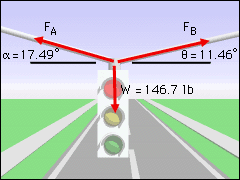Ch 1. Basics Multimedia Engineering Statics Problem Solving Units
 Chapter 1. Basics 2. Vectors 3. Forces 4. Moments 5. Rigid Bodies 6. Structures 7. Centroids/Inertia 8. Internal Loads 9. Friction 10. Work & Energy Appendix Basic Math Units Sections Search eBooks Dynamics Fluids Math Mechanics Statics Thermodynamics Author(s): Kurt Gramoll ©Kurt GramollSTATICS - CASE STUDY IntroductionProblem Graphic Susan, a new engineering student, is being very careful to write down each calculation step as she proceeds through a complex problem. Her friend Sam, on the other hand, wants to round all numbers to only two digits to get done faster.What is known: The traffic light weighs 146.7 lb. Cable A is 17.49° from the horizontal. Cable B is 11.46° from the horizontal. Questions What is the tension in both cables using four significant digits and two significant digits? Does rounding numbers to only two digits still provide an accurate answer? ApproachForce Diagram Solve the problem using the basic steps in engineering problem solving: 1) read 2) draw diagrams 3) write equations 4) solve and 5) check. For step 3, use the summation of forces in both the x and y directions:                   ΣFx = 0        ΣFy = 0 Solve twice, once with four significant digits and once with two significant digits.

Practice Homework and Test problems now available in the 'Eng Statics' mobile app
Includes over 500 problems with complete detailed solutions.
Available now at the Google Play Store and Apple App Store.